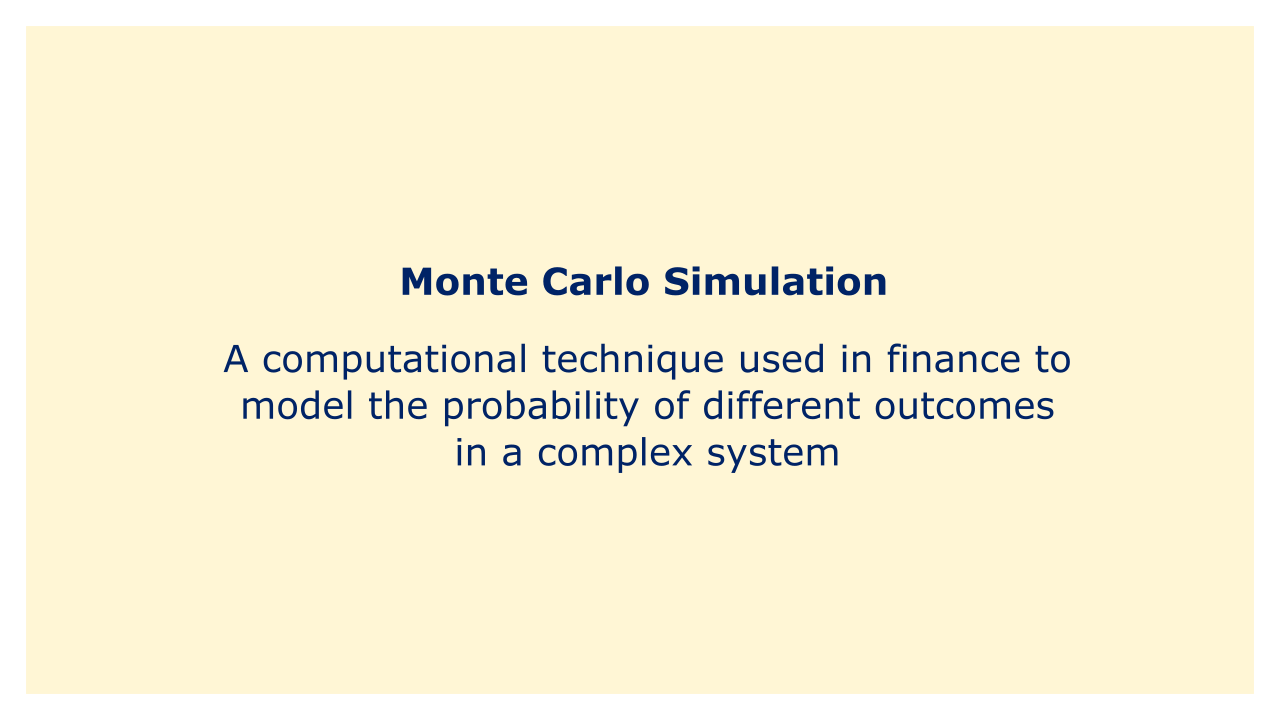# Monte Carlo SimulationImage: Moneybestpal.com

### A computational method known as Monte Carlo simulation is used in the field of finance to simulate the likelihood of various outcomes in complicated systems. It bears the name of a well-known casino in Monaco that is well-known for its slot machines.

A vast number of random trials are conducted in a Monte Carlo simulation to produce a variety of potential outcomes for a set of inputs or parameters. Based on the likelihood that each consequence will occur in the simulated trials, statistical methods are used in the simulation to estimate the probability of each occurrence.

In finance, Monte Carlo simulation is used to simulate the behavior of complex systems like financial markets, investment portfolios, or the value of an option or other derivative product. When the underlying system is influenced by numerous variables that are challenging to accurately anticipate or model, it is very helpful.

As an illustration, a Monte Carlo simulation of a stock portfolio might produce random returns for each stock in the portfolio based on historical data as well as additional inputs like interest rates, inflation, and market volatility. The simulation would then figure out the value of the portfolio for each simulated trial and use statistical techniques to assess the chance of various outcomes, such as the likelihood of a specific level of return or the possibility of a loss exceeding a particular threshold.

Monte Carlo simulation can be an effective tool for financial research because it enables analysts to more realistically and nuancedly analyze the behavior of complex systems and assess the likelihood of various outcomes than is possible with traditional mathematical models. The accuracy of the results, however, depends on the caliber of the inputs and assumptions employed in the Monte Carlo simulation, thus it is crucial to understand its limitations. Sensitivity analysis and scenario testing can be used to pinpoint important factors that influence outcomes and gauge how reliable the simulation results are.
Tags